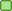###Author Topic: Tooltips/calltips showing wrong types  (Read 3113 times)

####oBFusCATed

• Developer
• Lives here!
•• Posts: 13392##### Tooltips/calltips showing wrong types
« on: January 31, 2015, 09:49:23 pm »
Here is the simple example.
Code
``#include <vector>int main(){    typedef std::vector<double> VEC;    VEC vec;    vec.push_back(1.5);    vec.push_back(2.5);    return 0;}``

Problems:
1. Hover over vec on lines 6, 7 or 8 and you'll see "VEC<double> main::vec" in the tooltip. This is obviously wrong it should be VEC main::vec or std::vector<double> main::vec.
2. Place the cursor over 1.5 or 2.5 and press ctrl-shift space to show the call tip. You'll see something like void std::vector::push_back(const value_type &x). This is obviously incomplete. It should either be std::vector<double>::push_... or VEC::push_...

And the biggest problem is that we show two different things in two separate UI components.
There is no consistency in the results...

Any hints how this could be fixed?

I'm running rev 10085 on linux, gcc 4.8.4 is the selected compiler.
(most of the time I ignore long posts)
[strangers don't send me private messages, I'll ignore them; post a topic in the forum, but first read the rules!]

####ollydbg##### Re: Tooltips/calltips showing wrong types
« Reply #1 on: February 01, 2015, 08:31:12 am »
I debugged for a while inside the function std::vector<CodeCompletion::CCToken> CodeCompletion::GetTokenAt(int pos, cbEditor* ed, bool& WXUNUSED(allowCallTip)), and for the case "VEC vec;"
In the function:
Code
``size_t NativeParserBase::ResolveExpression(TokenTree*                  tree,                                           std::queue<ParserComponent> components,                                           const TokenIdxSet&          searchScope,                                           TokenIdxSet&                result,                                           bool                        caseSense,                                           bool                        isPrefix)``
First, we know "vec" is a variable kind Token, and its type name is "VEC", this is done inside the function call:
Code
``        // e.g. A.BB.CCC.DDDD|        if (components.empty()) // is the last component (DDDD)            GenerateResultSet(tree, searchText, initialScope, initialResult, caseSense, isPrefix);        else // case sensitive and full-match always (A / BB / CCC)            GenerateResultSet(tree, searchText, initialScope, initialResult, true, false);``
Later, we need to give some disiplay name of this Token.
Code
``wxString Token::DisplayName() const{    wxString result;    if      (m_TokenKind == tkClass)        return result << _T("class ")     << m_Name << m_BaseArgs << _T(" {...}");    else if (m_TokenKind == tkNamespace)        return result << _T("namespace ") << m_Name << _T(" {...}");    else if (m_TokenKind == tkEnum)        return result << _T("enum ")      << m_Name << _T(" {...}");    else if (m_TokenKind == tkTypedef)    {        result << _T("typedef");        if (!m_FullType.IsEmpty())            result << _T(" ") << m_FullType;        if (result.Find('*', true) != wxNOT_FOUND)        {            result.RemoveLast();            return result << m_Name << _T(")") <<  GetFormattedArgs();        }        if (!m_TemplateArgument.IsEmpty())            result << m_TemplateArgument;        return result << _T(" ") << m_Name;    }    else if (m_TokenKind == tkMacroDef)    {        result << _T("#define ") << m_Name << GetFormattedArgs();        if (!m_FullType.IsEmpty())            result << _T(" ") << m_FullType;        return result;    }    // else    if (!m_FullType.IsEmpty())        result << m_FullType << m_TemplateArgument << _T(" ");    if (m_TokenKind == tkEnumerator)        return result << GetNamespace() << m_Name << _T("=") << GetFormattedArgs();    return result << GetNamespace() << m_Name << GetStrippedArgs();}``
The bad thing is that this Token's m_TemplateArgument = "<double>". So, we get the "VEC<double>...". Do need to always add this string inside DisplayName()?
If some piece of memory should be reused, turn them to variables (or const variables).
If some piece of operations should be reused, turn them to functions.
If they happened together, then turn them to classes.

####oBFusCATed

• Developer
• Lives here!
•• Posts: 13392##### Re: Tooltips/calltips showing wrong types
« Reply #2 on: February 01, 2015, 01:16:37 pm »
Probably we should show something like "VEC vec, where VEC = std::vector<double>". But I don't know it this is possible. And what happens if the there are more typedefs.
(most of the time I ignore long posts)
[strangers don't send me private messages, I'll ignore them; post a topic in the forum, but first read the rules!]